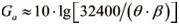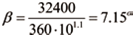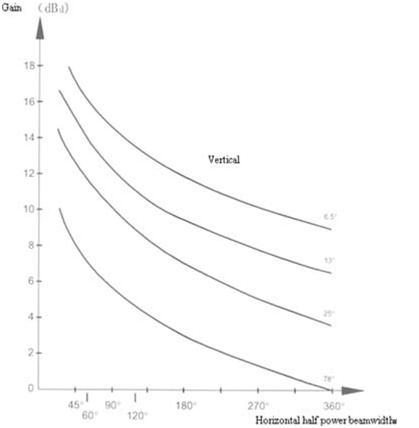# What is Beamwidth and Relation between Beamwidth and Gain

What is Beamwidth?

The beamwidth is also called the half power beamwidth, including horizontal beamwidth and vertical beamwidth. The horizontal beamwidth and vertical beamwidth is the beamwidth between two points where the power is lower 50% (3 dB) than the maximum radiation power. The common horizontal beamwidth of eNodeB antennas includes 360°, 90°, 65°, 60°, and 33°. The common vertical beamwidth of eNodeB antennas includes 6.5°, 7°, 10°, 13°, and 16°.

Relation between Beamwidth and Gain

The antenna concentrates power. It strengthens the power of a direction while reducing the power of other directions. You can usually reduce the horizontal beamwidth to strengthen the power of a direction. When the antenna gain is fixed, the horizontal beamwidth is in inverse proportion to the vertical beamwidth, with their relation as below:Wherein,

• Ga: the antenna gain in the unit of dBi.
• β: the vertical beamwidth in the unit of dBi.
• θ: the horizontal beamwidth in the unit of dBi.

According to the previous formula, if you have known the antenna gain and horizontal, you can calculate the vertical beamwidth.

For example, for an omnidirectional antenna, the gain is 11 dBi, the horizontal beamwidth is 360°, so the vertical beamwidth is calculated as below:Due to the deficiency of design and manufacturing process, the actual vertical beamwidth of omnidirectional antenna is usually smaller than the calculated result. The less difference between the two beamwidth, the better the antenna is designed.According to the figure above, when the antenna gain is low, the vertical beamwidth and horizontal beamwidth are usually large. When the antenna gain is high, the vertical beamwidth and horizontal beamwidth are usually small. In addition, the antenna gain depends on the number of oscillators. The larger the number of oscillators, the higher the gain is and the larger the aperture of antenna (the effective receiving area) is. For an omnidirectional antenna, if the antenna gain increases by 3 dB, the antenna length will double. Therefore, the antenna gain is usually within 11 dBi.